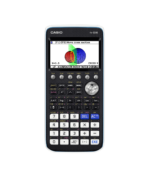Equations and Inequalities Archives - Casio Calculators

# Equations and Inequalities

Displaying Resources

FilterHow to Video
fx-CG50 A-Level GCSE IB
Quick Skill: Solving Equations with more than 1 Variable

How to use Solver to solve equations with more than one variable including SUVAT...How to Video
fx-CG50 A-Level GCSE IB
Quick Skill: Solving Linear Equations

How to solve a linear equation using the numerical SolveN function....Teach Leaflet
fx-CG50 A-Level
The Newton-Raphson Method

This resource guides learners to see graphically that the intersection with the ...Teach Leaflet
fx-CG50 A-Level
Solve Equations Using Fixed Point Iteration

This resource is an activity for solving equations numerically including the use...Teach Leaflet
fx-CG50 A-Level
Change of Sign Method for Finding Roots

This resource leads learners through the process of finding a root of a cubic eq...Teach Leaflet
fx-CG50 A-Level GCSE IB
Simultaneous Linear Equations

This learning resource consists of a learner worksheet to teach how to use the s...Teach Leaflet
fx-CG50 A-Level GCSE IB